Win up to 100% scholarship on Aakash BYJU'S JEE/NEET courses with ABNAT Win up to 100% scholarship on Aakash BYJU'S JEE/NEET courses with ABNAT

# JEE Main 2022 July 29 – Shift 1 Physics Question Paper with Solutions

The JEE Main 2022 July 29 – Shift 1 Physics Question Paper with Solutions is given on this page. Candidates are required to revise the JEE Main 2022 question paper with solutions so as to get an idea about the difficulty level of the JEE Main 2022 exam. JEE Main 2022 answer keys will help students to know the accurate solutions. Solving the JEE Main 2022 question papers is an effective way of JEE Main preparation. Find out the JEE Main 2022 July 29 – Shift 1 Physics Question Paper with Solutions given below.

## JEE Main 2022 29th July Shift 1 Physics Question Paper and Solutions

SECTION – A

Multiple Choice Questions: This section contains 20 multiple choice questions. Each question has 4 choices (1), (2), (3) and (4), out of which ONLY ONE is correct.

1. Given below are two statements: One is labelled as Assertion (A) and other is labelled as Reason (R).

Assertion (A) : Timeperiod of oscillation of a liquid drop depends on surf ace tension (S), ifdensity of the liquid is ρ and radius of the drop is r, then

$$\begin{array}{l} T=K\sqrt{\frac{\rho r^3}{S^{3/2}}}\end{array}$$
isdimensionally correct, where K is dimensionless.

Reason (R) :Using dimensional analysis we get R.H.S. having different dimension thanthat of time period.

In the light of above statements, choose the correct answer from the options given below.

(A) Both (A) and (R) are true and (R) is the correct explanation of (A)

(B) Both (A) and (R) are true but (R) is not thecorrect explanation of (A)

(C) (A) is true but (R) is false

(D) (A) is false but (R) is true

Sol.

$$\begin{array}{l} \left[\frac{\rho r^3}{T^{3/2}}\right]=\frac{\left[\text{ML}^{-3}\right]\left[\text{L}^3\right]}{\left[\text{ML}^0T^{-2}\right]^{3/2}}\neq \left[\text{T}\right]\end{array}$$

As the equation for first statement is wrong dimensionally.

⇒A is false and R is true.

2. A ball is thrown up vertically with a certain velocity so that, it reaches a maximum height h.Find the ratio of the times in which it is at height h/3 while going up and coming down respectively.

$$\begin{array}{l} (\text{A})\ \frac{\sqrt{2}-1}{\sqrt{2}+1} \end{array}$$
$$\begin{array}{l} (\text{B})\ \frac{\sqrt{3}-\sqrt{2}}{\sqrt{3}+\sqrt{2}}\end{array}$$
$$\begin{array}{l} (\text{C})\ \frac{\sqrt{3}-1}{\sqrt{3}+1}\end{array}$$
$$\begin{array}{l} (\text{D})\ \frac{1}{3}\end{array}$$

Sol.

$$\begin{array}{l} v=\sqrt{2gh}\end{array}$$
$$\begin{array}{l} \frac{h}{3}=\sqrt{2gh}t-\frac{1}{2}gt^2 \end{array}$$
$$\begin{array}{l} \frac{g}{2}t^2-\sqrt{2gh}t+\frac{h}{3}=0\end{array}$$
$$\begin{array}{l} \frac{t_1}{t_2}=\frac{\sqrt{2gh}+\sqrt{2gh-2gh/3}}{\sqrt{2gh}-\sqrt{2gh-2gh/3}}\end{array}$$
$$\begin{array}{l} =\frac{\sqrt{2}+\frac{2}{\sqrt{3}}}{\sqrt{2}-\frac{2}{\sqrt{3}}}=\frac{\sqrt{3}+\sqrt{2}}{\sqrt{3}-\sqrt{2}} \end{array}$$

3. If t = √x + 4, then

$$\begin{array}{l} \left(\frac{dx}{dt}\right)_{t=4}\end{array}$$
is

(A) 4

(B) Zero

(C) 8

(D) 16

Sol. x = (t – 4)2

$$\begin{array}{l} \frac{dx}{dt}=2t-8=0 \end{array}$$

4. A smooth circular groove has a smooth vertical wall as shown in figure. A block of mass m moves against the wall with a speed v. Which of the following curve represents the correct relation between the normal reaction on the block by the wall (N) and speed of the block (v)?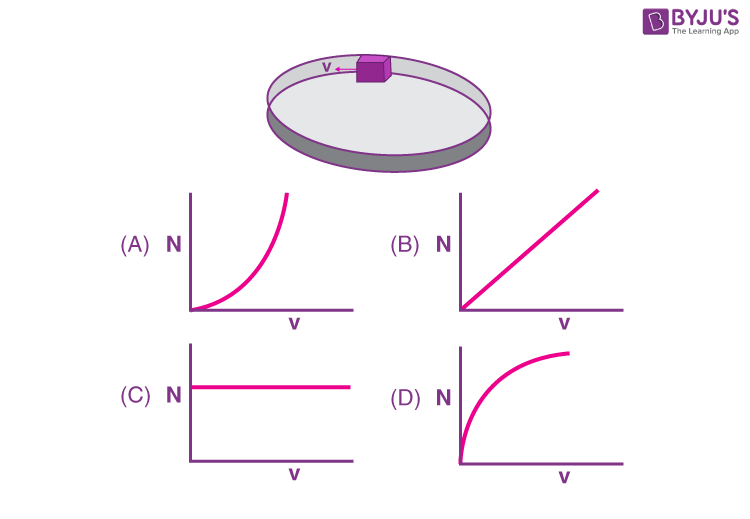Sol.

$$\begin{array}{l} N=\frac{mv^2}{r}\end{array}$$

⇒ Thegraph given in option A suits the best for the above relation.

5. A ball is projected with kinetic energy E, at an angle of 60° to the horizontal. The kinetic energy of this hall at the highest point of its flight will become

(A) Zero

$$\begin{array}{l} (\text{B})\ \frac{E}{2} \end{array}$$
$$\begin{array}{l} (\text{C})\ \frac{E}{4} \end{array}$$

(D) E

Sol.

$$\begin{array}{l} K.E.=E=\frac{1}{2}mv^2 \end{array}$$

at highest point

$$\begin{array}{l} K.E’=\frac{1}{2}mv^2\cos^2\theta\end{array}$$
$$\begin{array}{l} =\frac{1}{2}mv^2\left(\frac{1}{4}\right)\end{array}$$
$$\begin{array}{l} =\frac{E}{4} \end{array}$$

6. Two bodies of mass 1 kg and 3 kg have position vectors

$$\begin{array}{l} \hat{i}+2\hat{j}+\hat{k}\ \text{and}\ -3\hat{i}-2\hat{j}+\hat{k},\ \text{respectively}.\end{array}$$
The magnitude of position vector of centre of mass of this system will be similar to the magnitude of vector :

$$\begin{array}{l} (\text{A})\ \hat{i}+2\hat{j}+\hat{k}\end{array}$$
$$\begin{array}{l} (\text{B})\ -3\hat{i}-2\hat{j}+\hat{k}\end{array}$$
$$\begin{array}{l} (\text{C})\ -2\hat{j}+2\hat{k}\end{array}$$
$$\begin{array}{l} (\text{D})\ -2\hat{i}-\hat{j}+2\hat{k}\end{array}$$

Sol.

$$\begin{array}{l} \overline{r}_{com}=\frac{m_1\overline{r}_1+m_2\overline{r}_2}{m_1+m_2}\end{array}$$
$$\begin{array}{l} =\frac{\left(1-9\right)\hat{i}+\left(2-6\right)\hat{j}+\left(1+3\right)\hat{k}}{4} \end{array}$$
$$\begin{array}{l} =\frac{-8\hat{i}-4\hat{j}+4\hat{k}}{4} \end{array}$$
$$\begin{array}{l} \overline{r}_{com}=-2\hat{i}-\hat{j}+\hat{k}\end{array}$$
$$\begin{array}{l} \left|\overline{r}\right|=\sqrt{4+1+1}=\sqrt{6} \end{array}$$
$$\begin{array}{l} \left|\hat{i}+2\hat{j}+\hat{k}\right|=\sqrt{6} \end{array}$$

7. Given below are two statements: One is labelled as Assertion (A) and the other· is labelled asReason (R).

Assertion (A) :Clothes containing oil or grease stains cannot be cleaned by water wash.

Reason (R): Because the angle of contact between the oil/ grease and water is obtuse.

In the light of the above statements, choose the correct answer from the option given below.

(A) Both (A) and (R) are true and (R) is the correct explanation of (A)

(B) Both (A) and (R) are true but (R) is not the correct explanation of (A)

(C) (A) is true but (R) is false

(D) (A) is false but (R) is true

Sol. Due to obtuse angle of contact the water doesn’t wet the oiled surface properly and cannot wash it also.

⇒ Assertion is correct and Reason given is a correct explanation.

8. If the length of a wire is made double and radius is halved of its respective values. Then, theYoung’s modulus of the material of the wire will :

(A) Remain same

(B) Become 8 times its initial value

(C) Become ¼ th of its initial value

(D) Become 4 times its initial value

Sol. Young’s modulus of matter depends on material of wire and is independent of the dimensions of the wire. As the material remains same so Young’s modulus also remain same.

9. The time period of oscillation of a simple pendulum of length L suspended from the roof of avehicle, which moves without friction down an inclined plane of inclination α, is given by:

$$\begin{array}{l} (\text{A})\ 2\pi\sqrt{L/\left(g\cos\alpha\right)}\end{array}$$
$$\begin{array}{l} (\text{B})\ 2\pi\sqrt{L/\left(g\sin \alpha\right)} \end{array}$$
$$\begin{array}{l} (\text{C})\ 2\pi\sqrt{L/g} \end{array}$$
$$\begin{array}{l} (\text{D})\ 2\pi\sqrt{L/\left(g\tan\alpha\right)} \end{array}$$

Sol.

$$\begin{array}{l} \left|g_{eff}\right|=\left|\overline{g}-\overline{a}\right|\end{array}$$

geff = g cosθ

$$\begin{array}{l} \Rightarrow\ T=2\pi\sqrt{\frac{I}{g_{eff}}}\end{array}$$
$$\begin{array}{l} =2\pi\sqrt{\frac{L}{g\cos\theta} }\end{array}$$

10. A spherically symmetric charge distribution is considered with charge density varying as

$$\begin{array}{l} \rho\left(r\right)=\left\{\begin{matrix}\rho_0\left(\frac{3}{4}-\frac{r}{R}\right) & \text{for}~r\leq R \\\text{Zero} & \text{for}~r>R \\\end{matrix}\right.\end{array}$$

Where, r(r<R) is the distance from the centre O (as shown in figure) The electric field at point P will be :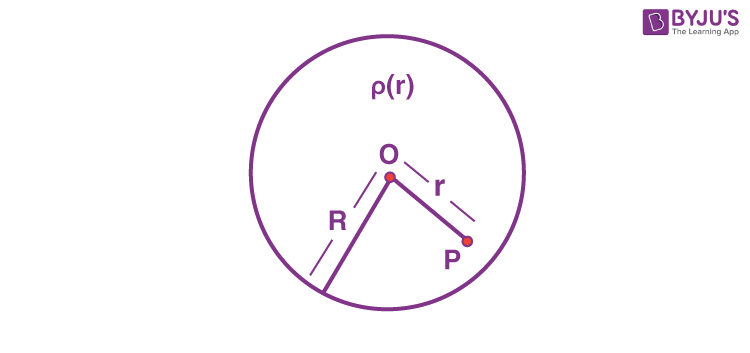$$\begin{array}{l} (\text{A})\ \frac{\rho_0r}{4{\varepsilon_0}}\left(\frac{3}{4}-\frac{r}{R}\right)\end{array}$$
$$\begin{array}{l} (\text{B})\ \frac{\rho_0r}{3{\varepsilon_0}}\left(\frac{3}{4}-\frac{r}{R}\right)\end{array}$$
$$\begin{array}{l} (\text{C})\ \frac{\rho_0r}{4{\varepsilon_0}}\left(1-\frac{r}{R}\right)\end{array}$$
$$\begin{array}{l} (\text{D})\ \frac{\rho_0 r}{5{\varepsilon_{0}}}\left(1-\frac{r}{R}\right)\end{array}$$

Sol.

$$\begin{array}{l} \left(4\pi r^2\right)E_\rho=\frac{Q_{in}}{\varepsilon_0}\end{array}$$
$$\begin{array}{l} =\frac{\int_0^r\rho_0 \left(\frac{3}{4}-\frac{r}{R}\right)4\pi r^2 dr}{\varepsilon_0} \end{array}$$
$$\begin{array}{l} =\frac{\rho_0\pi4}{\varepsilon_0}\left(\frac{r^3}{4}-\frac{r^4}{4R}\right)\end{array}$$
$$\begin{array}{l} E_\rho=\frac{\rho_0}{4\varepsilon_0}\left(r-\frac{r^2}{R}\right) \end{array}$$
$$\begin{array}{l} =\frac{\rho_0r}{4\varepsilon_0}\left(1-\frac{r}{R}\right)\end{array}$$

11. Given below are two statements.

Statement I : Electric potential is constant within and at the surface of each conductor.

Statement II : Electric field just outside a charged conductor is perpendicular to the surface of the conductor at every point.

In the light of the above statements, choose the most appropriate answer from the options given below.

(A) Both statement I and statement II are correct

(B) Both statement I and statement II are incorrect

(C) Statement I is correct but statement II is incorrect

(D) Statement I is incorrect but statement II is correct

Sol. Since

$$\begin{array}{l} \overrightarrow{E}_{\text{net}}=\overrightarrow{0}\end{array}$$
in the bulk of a conductor

⇒ Potential would be constant.

⇒ Statement I is correct.

$$\begin{array}{l}\text{Since a conductor’s surface is equipotential},\dot{E}\ \text{just outside is perpendicular to the surface}.\end{array}$$

12. Two metallic wires of identical dimensions are connected in series. If σ1 and σ2 are the conductivities of these wires, respectively, the effective conductivity of the combination is :

$$\begin{array}{l} (\text{A})\ \frac{\sigma_1\sigma_2}{\sigma_1+\sigma_2}\end{array}$$
$$\begin{array}{l} (\text{B})\ \frac{2\sigma_1\sigma_2}{\sigma_1+\sigma_2}\end{array}$$
$$\begin{array}{l} (\text{C})\ \frac{\sigma_1+\sigma_2}{2\sigma_1\sigma_2}\end{array}$$
$$\begin{array}{l} (\text{D})\ \frac{\sigma_1+\sigma_2}{\sigma_1\sigma_2}\end{array}$$

Sol. R = R1 + R2

$$\begin{array}{l} \Rightarrow\ \frac{I_1+I_2}{\sigma A}=\frac{I_1}{\sigma_1A}+\frac{I_2}{\sigma_2 A} \end{array}$$
$$\begin{array}{l} \Rightarrow\ \frac{2}{\sigma}=\frac{1}{\sigma_1}+\frac{1}{\sigma_2} \end{array}$$
$$\begin{array}{l} \Rightarrow\ \sigma = \frac{2\sigma_1\sigma_2}{\sigma_1+\sigma_2} \end{array}$$

13. An alternating emf E = 440 sin100πt is applied to a circuit containing an inductance of

$$\begin{array}{l} \frac{\sqrt{2}}{\pi}\text{H}.\ \text{If an a.c. ammeter is connected in the circuit, its reading will be}:\end{array}$$

(A) 4.4 A

(B) 1.55 A

(C) 2.2 A

(D) 3.11 A

Sol.

$$\begin{array}{l} I=\frac{V}{\omega L} \end{array}$$
$$\begin{array}{l} =\frac{440}{100\pi\times \frac{\sqrt{2}}{\pi}}=\frac{44}{10\sqrt{2}}\end{array}$$
$$\begin{array}{l} \Rightarrow\ I_{\text{rms}}=\frac{I}{\sqrt{2}}=\frac{44}{20}=2.2\text{A} \end{array}$$

14. A coil of inductance 1 H and resistance 100 Ω is connected to a battery of 6 V. Determine approximately :

(a) The time elapsed before the current acquires half of its steady – state value.

(b) The energy stored in the magnetic field associated with the coil at an instant 15 ms after the circuit is switched on.

(Given ln2 = 0.693, e–3/2 = 0.25)

(A) t = 10 ms; U = 2 mJ

(B) t = 10 ms; U = 1 mJ

(C) t = 7 ms; U = 1 mJ

(D) t = 7 ms; U = 2 mJ

Sol.

$$\begin{array}{l} i\left(t\right)=\frac{V}{R}\left(1-e^{-Rt/L}\right)\cdots\left(1\right)\end{array}$$
$$\begin{array}{l} \frac{L}{R}=\frac{1}{100}s\Rightarrow \frac{L}{R}=10\text{ ms}\cdots\left(2\right) \end{array}$$
$$\begin{array}{l} \frac{V}{2R}=\frac{V}{R}\left(1-e^{-Rt/L}\right)\end{array}$$
$$\begin{array}{l} \Rightarrow\ e^{-Rt/L}=\frac{1}{2}\Rightarrow t =\frac{L}{R}\text{ln }2=6.93\text{ ms} \end{array}$$
$$\begin{array}{l} U=\frac{1}{2}Li^2=\frac{1}{2}\left[1-e^{-15/10}\right]^2\left[\frac{6}{100}\right]^2\end{array}$$
$$\begin{array}{l} =\frac{1}{2}\left[1-0.25\right]^2\times 36\times 10^{-4}\end{array}$$

= 1 mJ

15. Match List-I with List-II:

List-I List-II
(a) UV rays (i) Diagnostic tool in medicine
(b) X-rays (ii) Water purification
(d) Infrared wave (iv) Improving visibility in foggy days

Choose the correct answer from the options given below :

(A) (a)-(iii), (b)-(ii), (c)-(i), (d)-(iv)

(B) (a)-(ii), (b)-(i), (c)-(iii), (d)-(iv)

(C) (a)-(ii), (b)-(iv), (c)-(iii), (d)-(i)

(D) (a)-(iii), (b)-(i), (c)-(ii), (d)-(iv)

Sol. UV – Water purification

X-rays – Diagnostic tool in medicine

Infrared wave – Improving visibility in foggy days.

16. The kinetic energy of emitted electron is E when the light incident on the metal has wavelength λ. To double the kinetic energy, the incident light must have wavelength :

$$\begin{array}{l} (\text{A})\ \frac{hc}{E\lambda-hc} \end{array}$$
$$\begin{array}{l} (\text{B})\ \frac{hc\lambda}{E\lambda+hc}\end{array}$$
$$\begin{array}{l} (\text{C})\ \frac{h\lambda}{E\lambda+hc}\end{array}$$
$$\begin{array}{l} (\text{D})\ \frac{hc\lambda}{E\lambda-hc}\end{array}$$

Sol.

$$\begin{array}{l} k=\frac{hc}{\lambda}-\phi=E\end{array}$$

and,

$$\begin{array}{l} 2k=\frac{hc}{\lambda_2}-\phi=2E\end{array}$$
$$\begin{array}{l} \Rightarrow\ \frac{hc}{\lambda}-E=\frac{hc}{\lambda_2}-2E \end{array}$$
$$\begin{array}{l} \Rightarrow\ \frac{hc}{\lambda_2}=\frac{hc}{\lambda}+E\end{array}$$
$$\begin{array}{l} \Rightarrow\ \lambda_2=\frac{hc\lambda}{hc+\lambda E}\end{array}$$

17. Find the ratio of energies of photons produced due to transition of an electron of hydrogen atom from its (i) second permitted energy level to the first level, and (ii) the highest permitted energy level to the first permitted level.

(A) 3 : 4

(B) 4 : 3

(C) 1 : 4

(D) 4 : 1

Sol.

$$\begin{array}{l} E_1=E_0\left(\frac{1}{1^2}-\frac{1}{2^2}\right)= E_0\times \frac{3}{4} \end{array}$$

E2 = E0

$$\begin{array}{l} \therefore\ \frac{E_1}{E_2}=\frac{3}{4} \end{array}$$

18. Find the modulation index of an AM wave having 8 V variation where maximum amplitude of the AM wave is 9 V.

(A) 0.8

(B) 0.5

(C) 0.2

(D) 0.1

Sol.

$$\begin{array}{l} \mu=\frac{\frac{8}{2}}{\left(9-\frac{8}{2}\right)}=\frac{4}{5}=0.8\end{array}$$

19. A travelling microscope has 20 divisions per cm on the main scale while its vernier scale has total 50 divisions and 25 vernier scale divisions are equal to 24 main scale divisions, what is the least count of the travelling microscope?

(A) 0.001 cm

(B) 0.002 mm

(C) 0.002 cm

(D) 0.005 cm

Sol.

$$\begin{array}{l} 1\text{{MSD}}=\frac{1}{20}\text{ cm}\end{array}$$
$$\begin{array}{l} 1\text{VSD}=\frac{24}{25}\times \frac{1}{20}\text{ cm}\end{array}$$

∴ Least count = 1 MSD – 1 VSD

$$\begin{array}{l} =\frac{1}{20}\left(1-\frac{24}{25}\right)\text{ cm}\end{array}$$
$$\begin{array}{l} =\frac{1}{20}\times \frac{1}{25}\text{ cm}\end{array}$$

= 0.002 cm

20. In an experiment to find out the diameter of the wire using a screw gauge, the following observations were noted :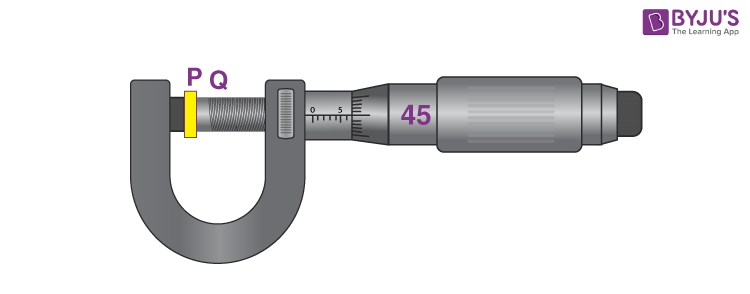(A) Screw moves 0.5 mm on main scale in one complete rotation

(B) Total divisions on circular scale = 50

(C) Main scale reading is 2.5 mm

(D) 45th division of circular scale is in the pitch line

(E) Instrument has 0.03 mm negative error

Then the diameter of wire is :

(A) 2.92 mm

(B) 2.54 mm

(C) 2.98 mm

(D) 3.45 mm

Sol.

$$\begin{array}{l} \text{L.C.}=\frac{0.5}{50}\text{ mm}=0.01\text{ mm}\end{array}$$

d = (2.5 + 45 × 0.01 + 0.03) mm

= 2.98

SECTION – B

Numerical Value Type Questions: This section contains 10 questions. In Section B, attempt any five questions out of 10. The answer to each question is a NUMERICAL VALUE. For each question, enter the correct numerical value (in decimal notation, truncated/rounded-off to the second decimal place; e.g. 06.25, 07.00, –00.33, –00.30, 30.27, –27.30) using the mouse and the on-screen virtual numeric keypad in the place designated to enter the answer.

1. An object is projected in the air with initial velocityu at an angle θ. The projectile motion is such that the horizontal range R, is maximum. Another object is projected in the air with a horizontal range half of the range of first object. The initial velocity remains same in both the case. The value of the angle of projection, at which the second object is projected, will be ______ degree.

Sol. θ = 45°

$$\begin{array}{l} R_1=\frac{R}{2}\end{array}$$
$$\begin{array}{l} \frac{u^2\sin2\theta_1}{g}=\frac{u^2\sin\left(90^\circ\right)}{2g} \end{array}$$

1 = 30°

θ1 = 15°

2. If the acceleration due to gravity experienced by a point mass at a height h above the surface of earth is same as that of the acceleration due to gravity at a depth αh(h<<Re) from the earth surface. The value of α will be ________.

(Use Re=6400 km)

Sol.

$$\begin{array}{l} g\left(1-\frac{2h}{R}\right)=g\left(1-\frac{d}{R}\right)\end{array}$$

⇒ 2h = d

⇒ α = 2

3. The pressure P1 and density d1 of diatomic gas (γ = 7/5) changes suddenly to P2 (>P1) and d2 respectively during an adiabatic process.The temperature of the gas increases and becomes _____ times of its initial temperature.

(Given d2/d1 =32)

Sol.

$$\begin{array}{l} P_1V_1^\gamma=P_2V_2^2\end{array}$$
$$\begin{array}{l} \frac{P_1}{d_1^\gamma}=\frac{P_2}{d_2^\gamma} \end{array}$$
$$\begin{array}{l} \frac{d_1T_1}{d_1^\gamma}=\frac{d_2T_2}{d_2^\gamma}\end{array}$$
$$\begin{array}{l} T_2=\left(\frac{d_2}{d_1}\right)^{\gamma-1}T_1 \end{array}$$
$$\begin{array}{l} =\left(32\right)^\frac{2}{5}T_1\end{array}$$

T2 = 4T1

4. One mole of a monoatomic gas is mixed with three moles of a diatomic gas. The molecular specific heat of mixture at constant volume is

$$\begin{array}{l} \frac{\alpha^2}{4}R\ J/mol K;\end{array}$$
then the value of α will be _______.

(Assume that the given diatomic gas has no vibrational mode).

Sol.

$$\begin{array}{l} C_V=\frac{f}{2}R \end{array}$$

Total degree of freedoms

= 1 × 3 + 3 × 5 = 18

$$\begin{array}{l} \frac{\alpha^2}{4}=\frac{18}{2n}=\frac{18}{2\times 4} \end{array}$$

⇒ α2 = 9

α = 3

5. The current I flowing through the given circuit will be ________A.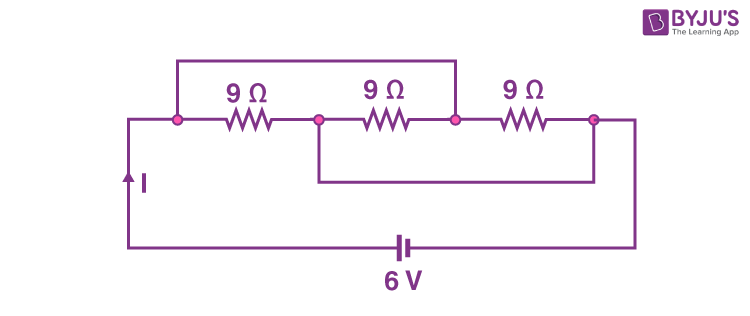Sol. All 9 Ω resistances are in parallel

Req = 3 Ω

$$\begin{array}{l} I=\frac{6}{3}A=2A\end{array}$$

6. A closely wounded circular coil of radius 5 cm produces a magnetic field of 37.68×10–4 T at its center. The current through the coil is _______ A.

[Given, number of turns in the coil is 100 and π =3.14]

Sol.

$$\begin{array}{l} B=\frac{\mu_0nI}{2R} \end{array}$$
$$\begin{array}{l} 37.68\times 10^{-4}=\frac{4\pi\times10^{-7}100I}{2\times5\times10^{-2}}\end{array}$$
$$\begin{array}{l} I=\frac{300A}{100} \end{array}$$

= 3A

7. Two light beams of intensities 4I and 9I interfere on a screen. The phase difference between these beams on the screen at point A is zero and at point B is π. The difference of resultant intensities, at the point A and B, will be_______I.

Sol.

$$\begin{array}{l} I_A=\left(\sqrt{I_1}+\sqrt{I_2}\right)^2=25I\end{array}$$
$$\begin{array}{l} I_B=\left(\sqrt{I_1}-\sqrt{I_2}\right)^2=I\end{array}$$

So,IA IB = 24I

8. A wire of length 314 cm carrying current of 14 A is bent to form a circle. The magnetic moment of the coil is _______ A–m2. [Given π =3.14]

Sol.

$$\begin{array}{l} R=\frac{I}{2\pi}=\frac{314}{2\times3.14}=50\text{ cm} \end{array}$$

μ = πR2i

= 14 × 3.14 × (0.5)2

= 11 A–m2

9. The XY plane be taken as the boundary between two transparent media M1 and M2.M1 in Z 0 has a refractive index of √2 and M2 with Z<0 has a refractive index of √3. A ray of light travelling in M1 along the direction given by the vector

$$\begin{array}{l} \overrightarrow{P}=4\sqrt{3}\hat{i}-3\sqrt{3}\hat{j}-5\hat{k}, \end{array}$$
is incident on the plane of separation. The value of difference between the angle of incident in M1 and the angle of refraction in M2 will be ______ degree.

Sol. Normal will be

$$\begin{array}{l} -\hat{k} \end{array}$$
so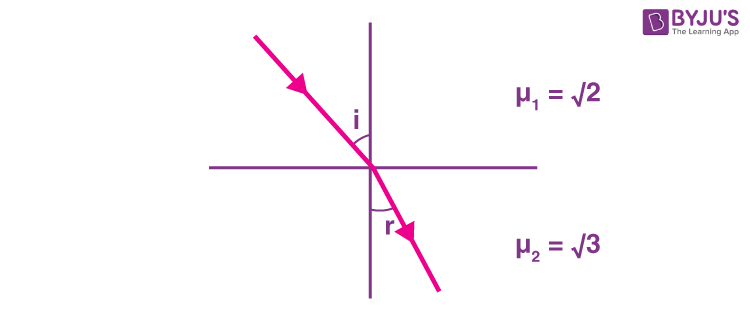$$\begin{array}{l} \cos i=\frac{\overleftarrow{P}\cdot \hat{n}}{\left|\overleftarrow{P}\right|\cdot\left|\hat{n}\right|}\end{array}$$
$$\begin{array}{l} \frac{5}{10}=\frac{1}{2}\end{array}$$

i = 60°

and using snells law

$$\begin{array}{l} \sqrt{2}\sin60^\circ=\sqrt{3}\sin r\end{array}$$
$$\begin{array}{l} \frac{\sqrt{3}}{\sqrt{2}}=\sqrt{3}\sin r\end{array}$$

r = 45°

So, ir = 15°

10. If the potential barrier across a pn junction is 0.6 V. Then the electric field intensity, in the depletion region having the width of 6×10–6 m, will be ______× 105 N/C.

Sol.

$$\begin{array}{l} E=\frac{V}{d}=\frac{0.6}{6\times10^{-6}}=1\times 10^5\end{array}$$

### Download PDF of JEE Main 2022 July 29 Shift 1 Physics Paper & Solutions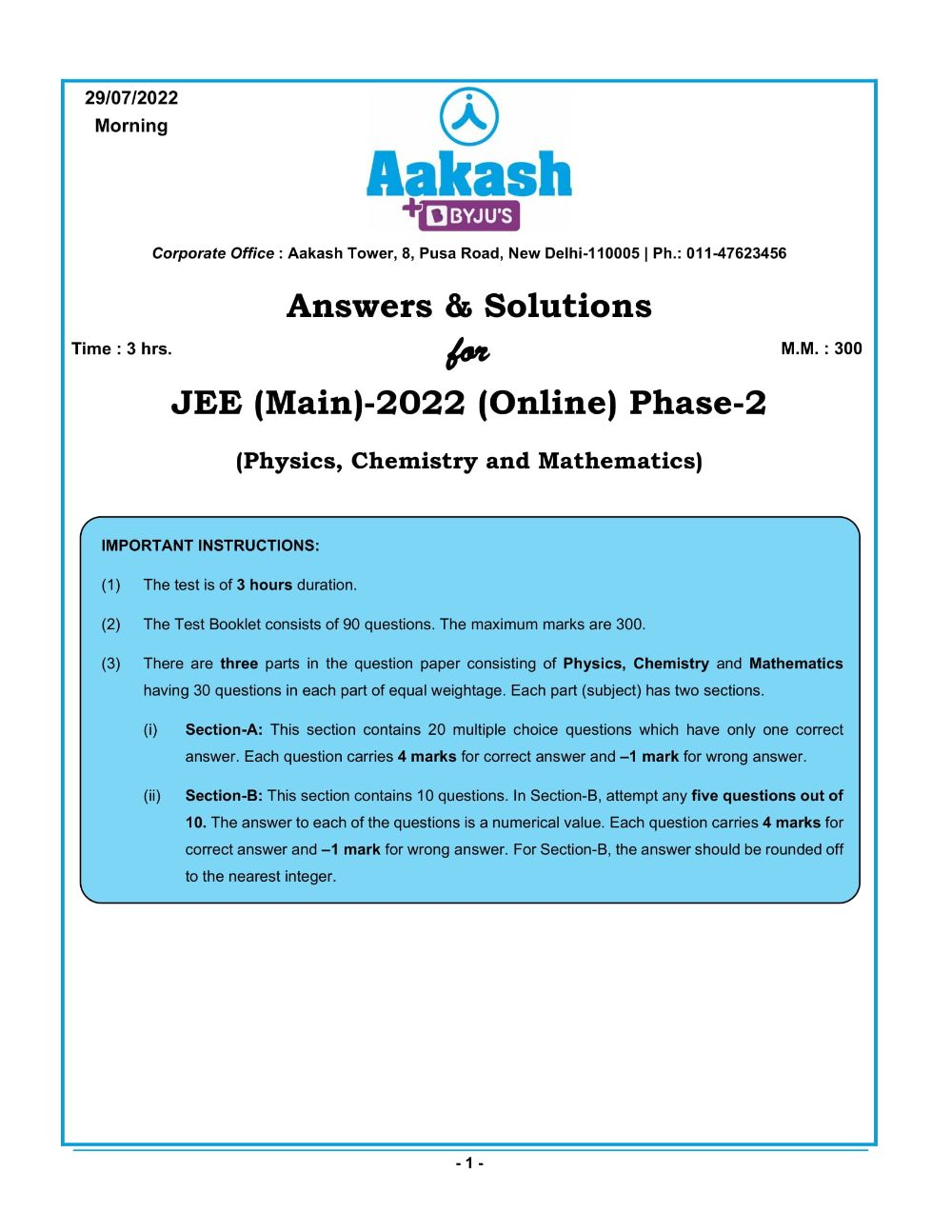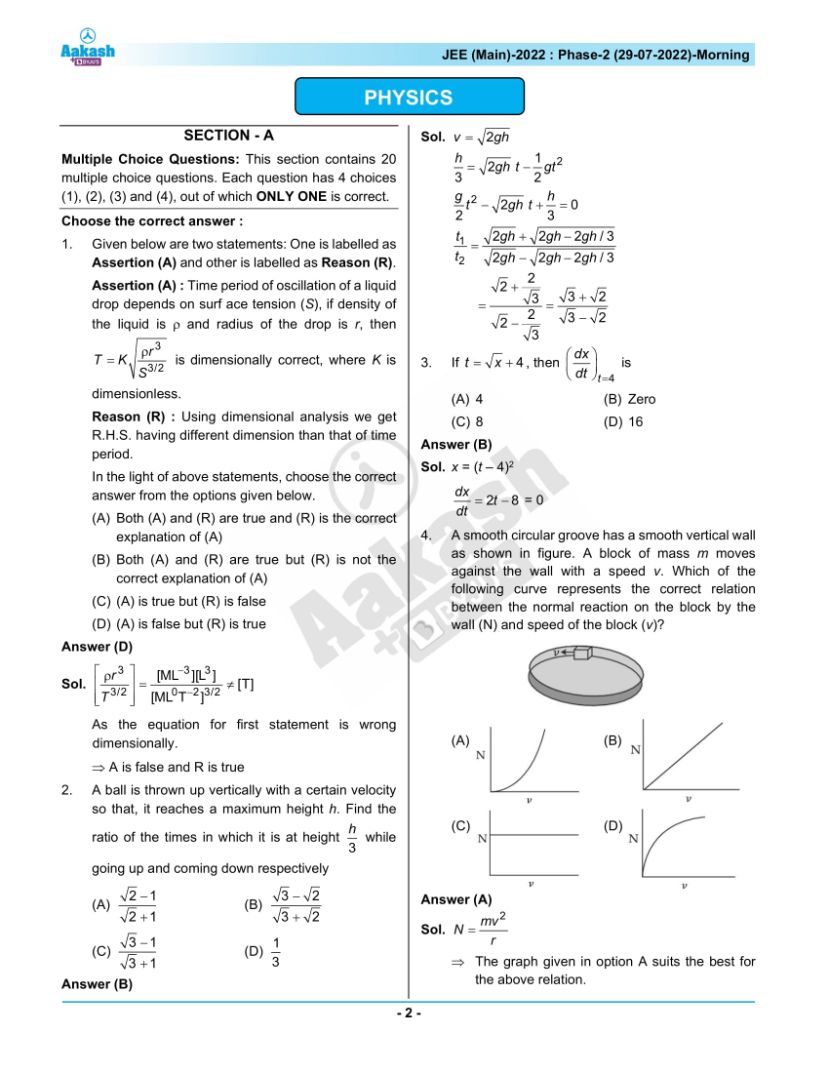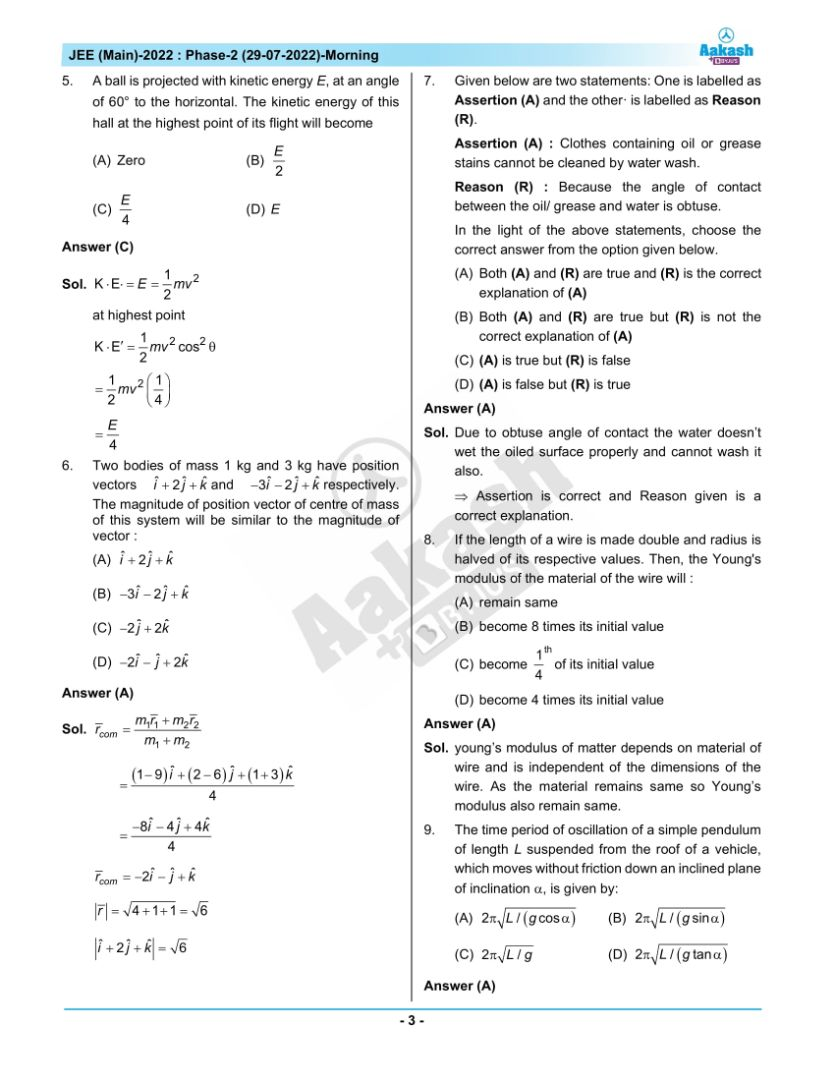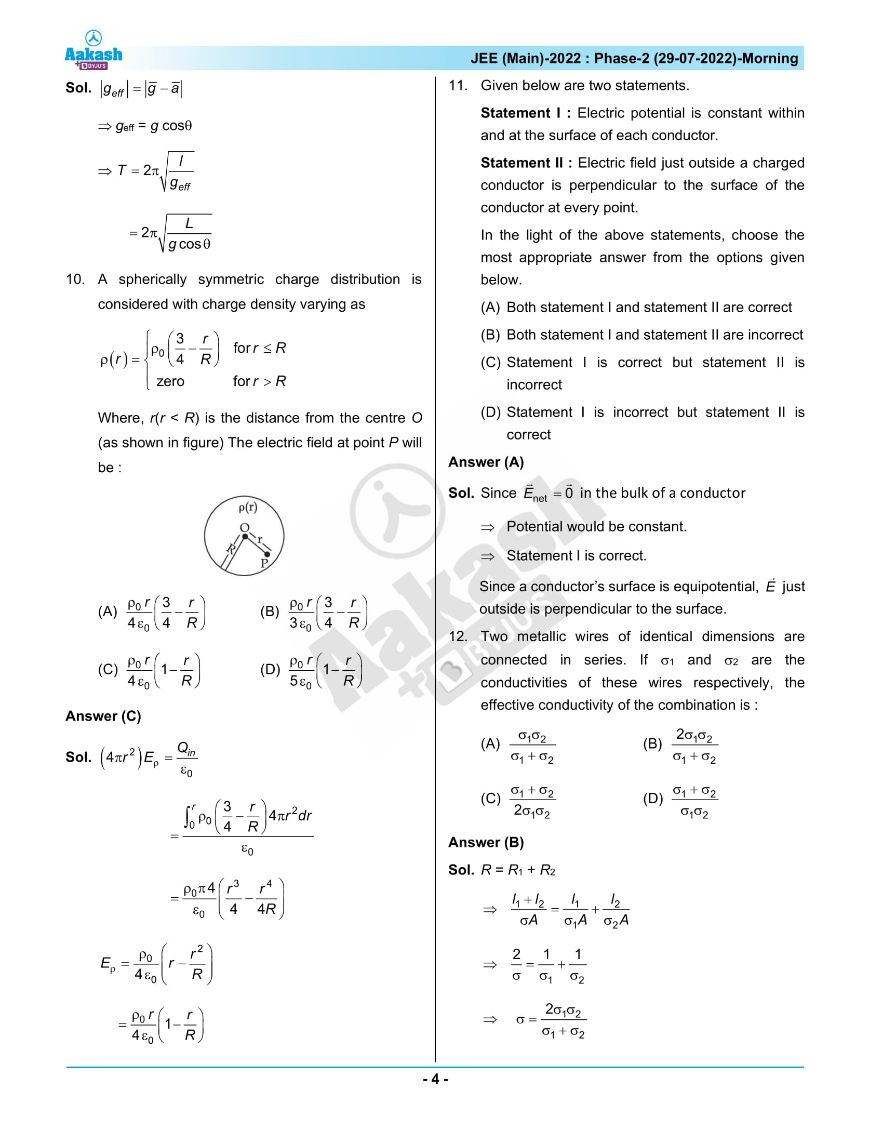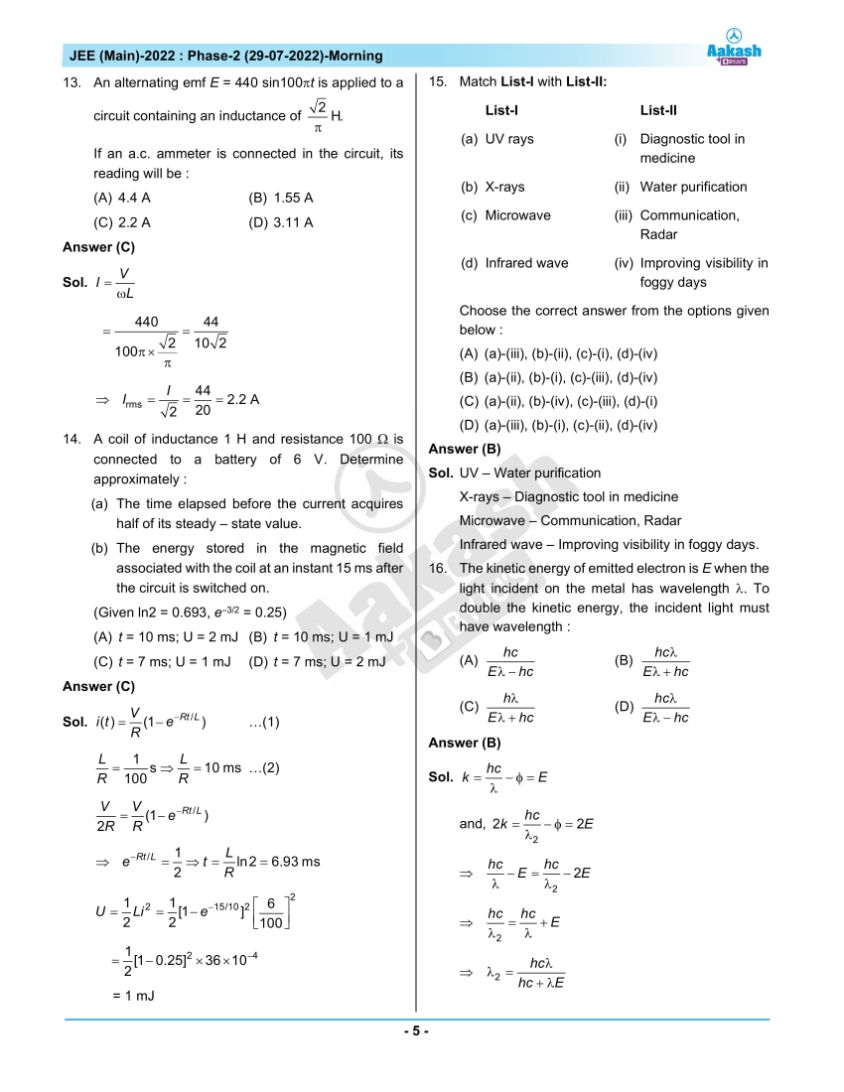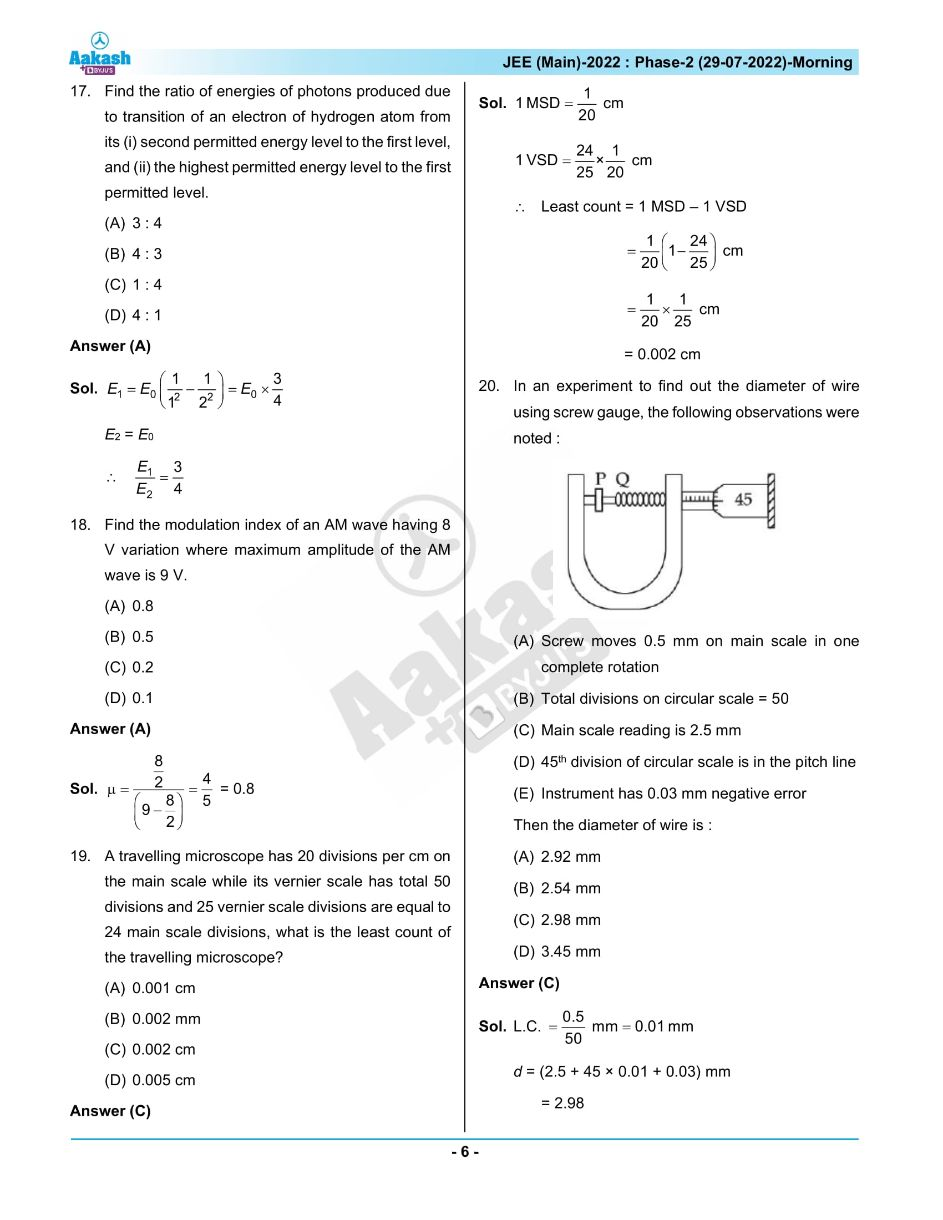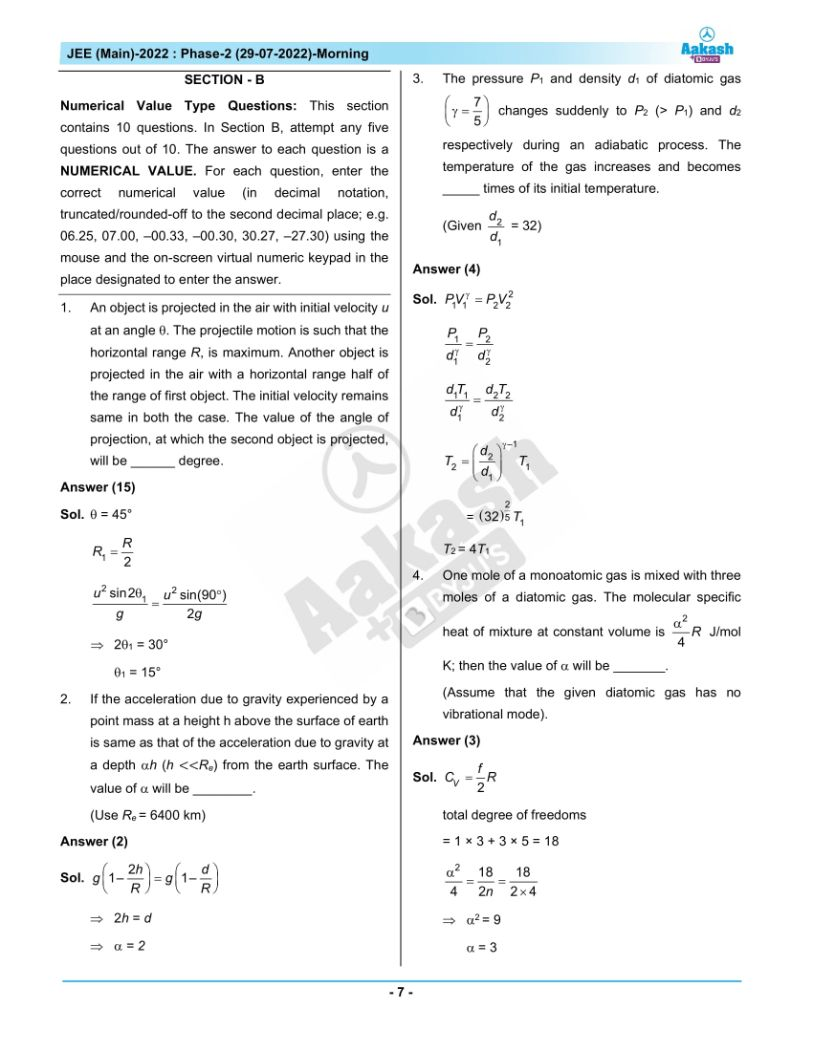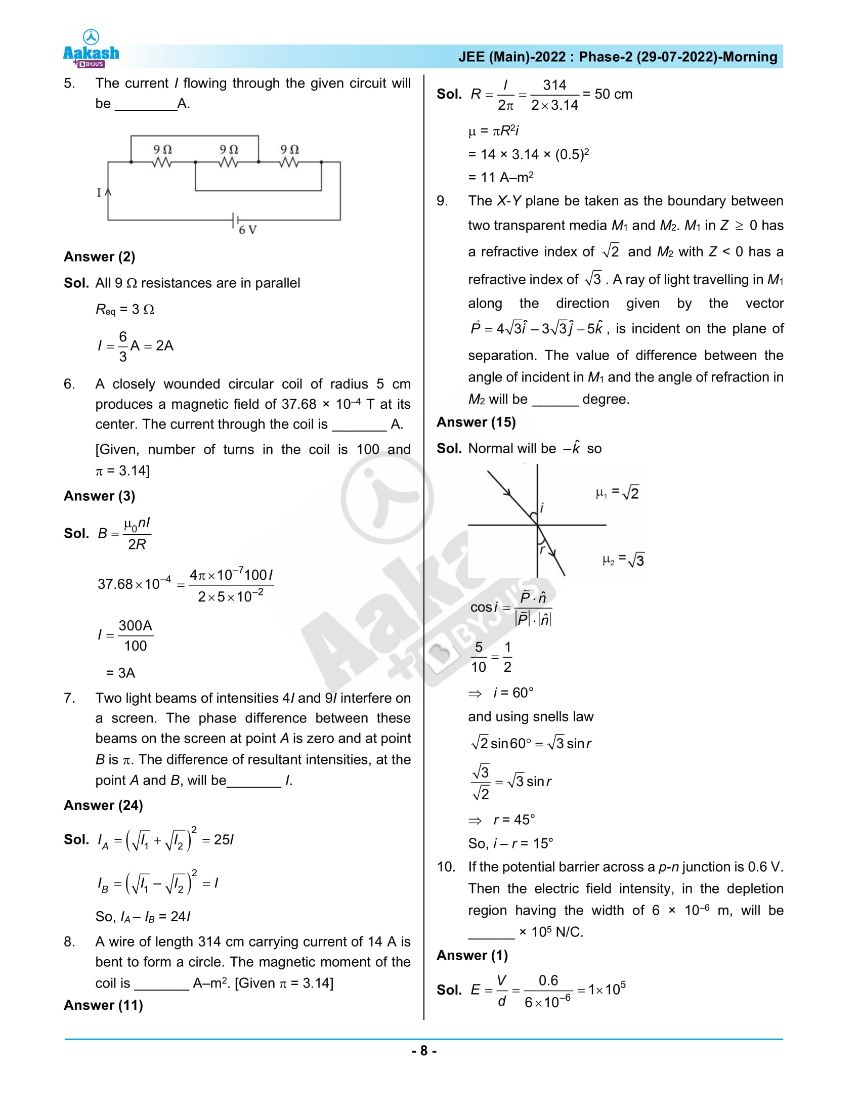## JEE Main 2022 July 29th Shift 1 Paper Analysis